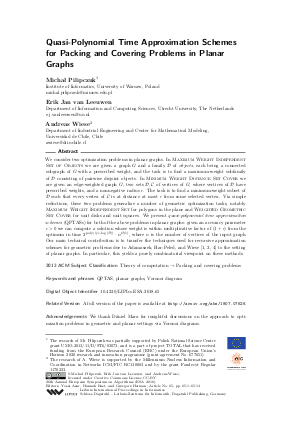Document# Quasi-Polynomial Time Approximation Schemes for Packing and Covering Problems in Planar Graphs

### Authors Michal Pilipczuk, Erik Jan van Leeuwen, Andreas Wiese## File

LIPIcs.ESA.2018.65.pdf
• Filesize: 0.69 MB
• 13 pages

## Cite As

Michal Pilipczuk, Erik Jan van Leeuwen, and Andreas Wiese. Quasi-Polynomial Time Approximation Schemes for Packing and Covering Problems in Planar Graphs. In 26th Annual European Symposium on Algorithms (ESA 2018). Leibniz International Proceedings in Informatics (LIPIcs), Volume 112, pp. 65:1-65:13, Schloss Dagstuhl - Leibniz-Zentrum für Informatik (2018)
https://doi.org/10.4230/LIPIcs.ESA.2018.65

## Abstract

We consider two optimization problems in planar graphs. In {Maximum Weight Independent Set of Objects} we are given a graph G and a family D of {objects}, each being a connected subgraph of G with a prescribed weight, and the task is to find a maximum-weight subfamily of D consisting of pairwise disjoint objects. In {Minimum Weight Distance Set Cover} we are given an edge-weighted graph G, two sets D,C of vertices of G, where vertices of D have prescribed weights, and a nonnegative radius r. The task is to find a minimum-weight subset of D such that every vertex of C is at distance at most r from some selected vertex. Via simple reductions, these two problems generalize a number of geometric optimization tasks, notably {Maximum Weight Independent Set} for polygons in the plane and {Weighted Geometric Set Cover} for unit disks and unit squares. We present {quasi-polynomial time approximation schemes} (QPTASs) for both of the above problems in planar graphs: given an accuracy parameter epsilon>0 we can compute a solution whose weight is within multiplicative factor of (1+epsilon) from the optimum in time 2^{poly(1/epsilon,log |D|)}* n^{O(1)}, where n is the number of vertices of the input graph. Our main technical contribution is to transfer the techniques used for recursive approximation schemes for geometric problems due to Adamaszek, Har-Peled, and Wiese [Adamaszek and Wiese, 2013; Adamaszek and Wiese, 2014; Sariel Har-Peled, 2014] to the setting of planar graphs. In particular, this yields a purely combinatorial viewpoint on these methods.

## Subject Classification

##### ACM Subject Classification
• Theory of computation → Packing and covering problems
##### Keywords
• QPTAS
• planar graphs
• Voronoi diagram

## Metrics

• Access Statistics
• Total Accesses (updated on a weekly basis)
0

## References

1. Anna Adamaszek and Andreas Wiese. Approximation schemes for maximum weight independent set of rectangles. In Proc. FOCS 2013, pages 400-409. IEEE, 2013.2. Anna Adamaszek and Andreas Wiese. A QPTAS for maximum weight independent set of polygons with polylogarithmically many vertices. In Proc. SODA 2014, pages 645-656. SIAM, 2014.3. Qian-Ping Gu and Hisao Tamaki. Improved bounds on the planar branchwidth with respect to the largest grid minor size. Algorithmica, 64(3):416-453, 2012.4. Sariel Har-Peled. Quasi-polynomial time approximation scheme for sparse subsets of polygons. In Proc. SoCG 2014, pages 120-129. SIAM, 2014.5. Dániel Marx and Michał Pilipczuk. Optimal parameterized algorithms for planar facility location problems using Voronoi diagrams. In Proc. ESA 2015, volume 9294 of LNCS, pages 865-877. Springer, 2015.6. Gary L. Miller. Finding small simple cycle separators for 2-connected planar graphs. J. Comput. Syst. Sci., 32(3):265-279, 1986.7. Nabil H. Mustafa, Rajiv Raman, and Saurabh Ray. Quasi-polynomial time approximation scheme for weighted geometric set cover on pseudodisks and halfspaces. SIAM J. Comput., 44(6):1650-1669, 2015.8. Paul D. Seymour and Robin Thomas. Call routing and the ratcatcher. Combinatorica, 14(2):217-241, 1994.X

Feedback for Dagstuhl Publishing